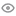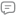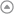New update is available. Click here to update.I am Graduate in mechanical engineering and being my interest toward to technology i willing the full Stack Web Development . As the technology increase decide to movie the technological sector that w...Godavari College of Engineering 2022C++ - Default language
My StatsEXP gained4666Level
5 (Champion)
Community statsDiscussions
12
Know more168
Total problems solved
152
Easy
12
Moderate
4
Hard
0
NinjaCurrent streak:

0 days

Longest streak:

3 days

Less

More

Achievements7
Ronin
Topics
Sorting
Math
+ 4 more6
Samurai
Topics
Arrays
Strings
Heap
+ 3 more1
Sensei
Topics
Recursion
Discussions
c++
Interview problems

/*    Time complexity: O(N ^ 2)    Space complexity: O(1)

Where 'N' represents the size of given array. */

#include<climits>

int findGreater(vector<int> &arr, int value, int from, int to) {    int min = INT_MAX;    int minIndex = -1;

for (int i = from; i < to; i++)    {        if (arr[i] == value)        {            return i;        }

if (arr[i] > value && min > arr[i])        {            min = arr[i];            minIndex = i;        }    }

return minIndex; }

int findSmaller(vector<int> &arr, int value, int from, int to) {    int max = INT_MIN;    int maxIndex = -1;

for (int i = from; i < to; i++)    {        if (arr[i] == value)        {            return i;        }

if (arr[i] < value && max < arr[i])        {            max = arr[i];            maxIndex = i;        }    }

return maxIndex; }

int ninjaJump(vector<int> &arr, int n) {    if (n < 1)    {        return 0;    }

int ans = 0;

for (int i = 0; i < n; i++)    {                // If we reached the end we exit from the loop.        if (i == n - 1)        {            ans++;            break;        }

int value = arr[i];        int index = i;

while (index < n)        {            value = arr[index];                        // We are calling this function to find greater element index.            index = findGreater(arr, value, index + 1, n);

if (index == n - 1)            {                ans++;                break;            }            else if (index == -1)            {                break;            }

value = arr[index];                        // We are calling this function to find smaller element index.            index = findSmaller(arr, value, index + 1, n);

if (index == n - 1)            {                ans++;                break;            }            else if (index == -1)            {                break;            }        }     }        return ans; }

msmane112
Published On 13-Aug-2023132 views0 repliescode for python
Interview problems

'''    Time Complexity : O(N)    Space Complexity : O(1)

where 'N' is the number of piles. '''

from typing import *

def ninjaGame(a: List[int], n: int)-> int:

ans = 0

# Calculate the 'Nim-Sum'.    for i in range(n):        ans ^= a[i]

# If 'Nim-Sum' is non-zero, Ninja wins.    if ans != 0:        return 1

# Else, Ninja loses.    return 0

msmane112
Published On 13-Aug-2023116 views0 repliesInterview problems

'''     Time Complexity: O(N)    Space Complexity: O(1)

where N is the number of nodes in the Linked List. '''

#   List Node Class. class Node:    def __init__(self, data):

self.data = data        self.next = None

#   Dummy nodes for two sub-lists.    head1 = Node(-1)    head2 = Node(-1)

#   Pointers to track the last node of each sub-lists.    tail1 = head1    tail2 = head2

#   To track at which sub-list we have to append.    listNumber = 0

while(head != None):        if(not listNumber):            tail1.next = head            tail1 = tail1.next        else:            tail2.next = head            tail2 = tail2.next

#   Swap sub-lists.        listNumber ^= 1

#   Append NULL at both sub-lists.    tail1.next = None    tail2.next = None

msmane112
Published On 13-Aug-202344 views0 repliespython solution for given q. but need muv=ch better time complexity
Interview problems

''' Time complexity: O(3^N) Space Complexity: O(N) Where N is the length of strings to be found. '''

MOD = 1000000007

def countStringsHelper(m, freqB, freqC):

if (freqB > 1 or freqC > 2):

# Invalid string.        return 0

if (m == 0):

# Valid string generated.        return 1

if (freqB == 1 and freqC == 2):

# Only one string is possible i.e. string with all remaining characters as ‘a’.        return 1

# One by one choosing 'a', 'b' and 'c' as the next character.    counter = countStringsHelper(m - 1, freqB, freqC)    counter = (counter + countStringsHelper(m - 1, freqB + 1, freqC)) % MOD    counter = (counter + countStringsHelper(m - 1, freqB, freqC + 1)) % MOD

return counter

def countStrings(n):

return countStringsHelper(n, 0, 0)

msmane112
Published On 14-May-202392 views0 replies